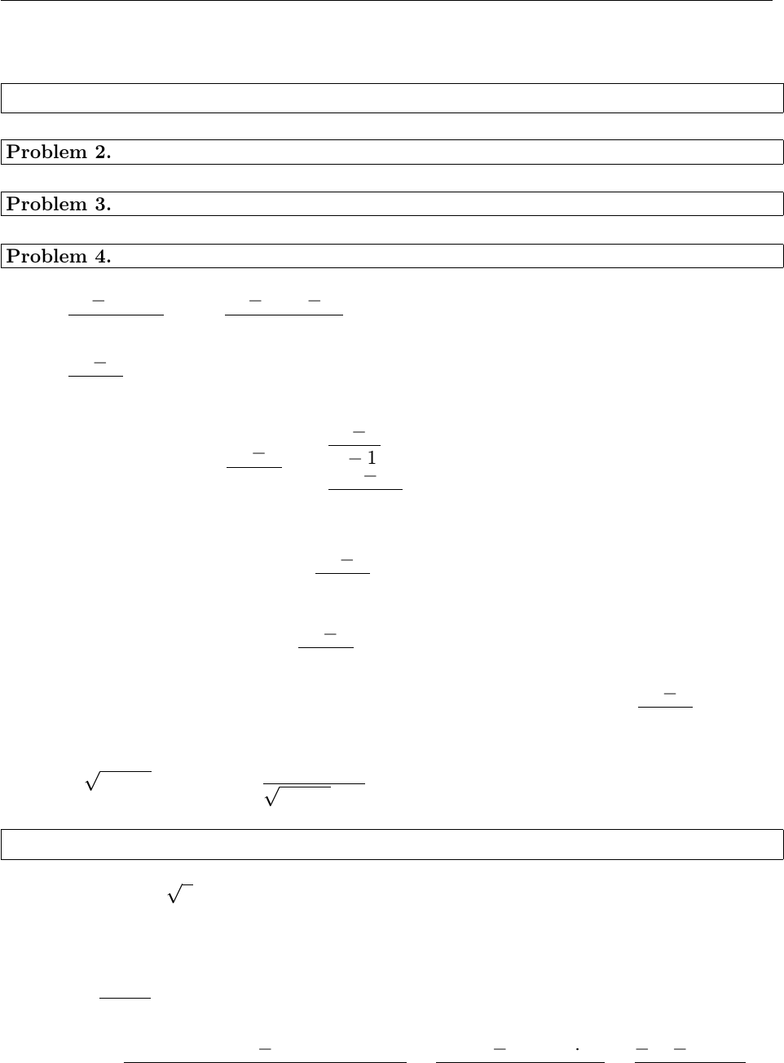# MATH V1101 Study Guide - Midterm Guide: Quotient Rule, Power Rule, 32X

13 views3 pages
School
Columbia University
Department
Mathematics
Course
MATH V1101
ProfessorMath V1101: Calculus I (Chao Li) Midterm I (Solutions to sample and practice problems)
Sample A
Problem 1. (8 points) Mark True or False. No justiﬁcation is needed.
FFTF FTFF
Problem 2.
b, a, c, d, e
Problem 3.
b, e, a, d, c
Problem 4.
(1) lim
x1
x24x+ 3
x1= lim
x1
(x3)(x1)
x1= lim
x1(x3) = 2.
(2) lim
x1
x21
|x1|does not exist. In fact,
x21
|x1|=
x21
x1=x+ 1, x > 1,
x21
(x1) =(x+ 1), x < 1.
So
lim
x1+
x21
|x1|= lim
x1+(x+ 1) = 2,
and
lim
x1
x21
|x1|= lim
x1(x+ 1) = 2.
The two one-sided limits exist but they are not the same, therefore lim
x1
x21
|x1|does not
exist.
(3) lim
x+
x2+ 4 x= lim
x+
4
x2+ 4 + x= 0.
Problem 5. (Derivatives)
(1) f(x) = x2(1 2x) = x22x5/2, using the power rule we get
f(x) = 2x2(5/2·x3/2) = 2x5x3/2.
(2) f(x) = x+ 3
x2+ 1. Using the quotient rule we get
f(x) = (x+ 3)(x2+ 1) (x+ 3)(x2+ 1)
(x2+ 1)2=x2+ 1 (x+ 3) ·2x
(x2+ 1)2=x26x+ 1
(x2+ 1)2.
1
Unlock document

This preview shows page 1 of the document.
Unlock all 3 pages and 3 million more documents.